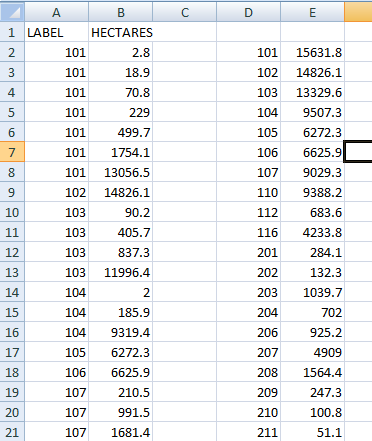# A20.5 Excel: Conditional sum - LUPMISManual

Go to content

Annexes 11-20 > A20. Various IT advices

A20.5 Excel: Conditional Sum

Level of expertise required for this Chapter: Intermediate

For a GIS crosstabulation to produce statistics how large is the overlay of different map with more than one unit, you should be familiar with the Excel conditional sum command. This is similar to the the Frequency command, explained in Annex 20.2, but it totals up all figures (e.g. areas).

As explained in Chapter 5.6.3 ('Polygons per polygons'), you can overlay polygons on polygons and create a table, which lists all polygons overlaid on the other polygons (e.g. District number). You want to know how many sqkm are in each polygon (District).

1. From Map Maker you have converted this file to Excel: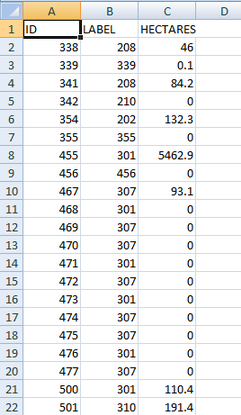1. Delete column A, as you are not interested in the GIS ids. Then copy all labels from new column A into a new column (here: D):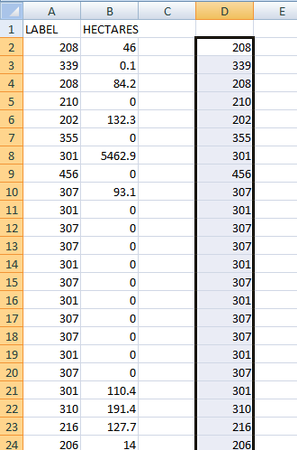2. Sort this new column:
Select all values in this column (D) > Data > Sort > Sort by this column > Order: Smallest to Largest > OK, keep it selected and remove duplicates: Data > Remove duplicates: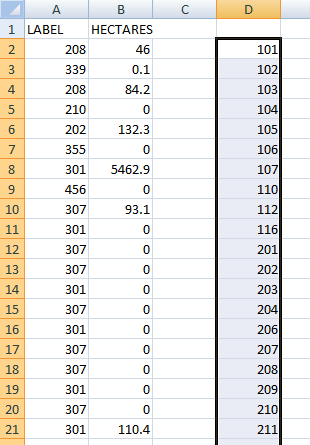3. In the cell next to the top data line (here: E2), invoke the data function SUMIF: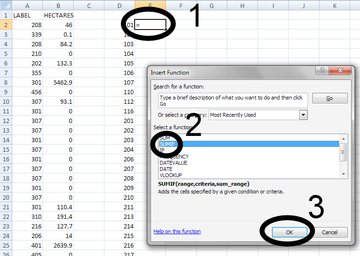and define the range (4: leftmost colum), criteria (5: D2) and the sum_range (6: column next to the range), and press OK (7):4. The first data row is calculated. Before you copy this command down to all, set the \$ as row-fixer in front of all row numbers), then copy the command down:5. The result is an Excel file with all added figures (e.g. areas) for each polygon: